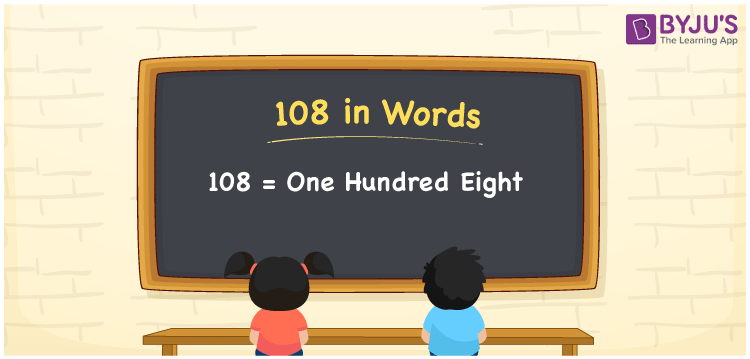# 108 in words

108 in words is written as One Hundred and Eight. 108 represents the count or value. The article on Counting Numbers can give you an idea about counting. The number 108 is used in expressions that relate to money, days, distance, length, weight and more. Let us consider an example for 108. “I performed 108 poses on International Yoga Day.” Another example is, “The competition required to have One Hundred and Eight worded passages.”

 108 in words One Hundred and Eight One Hundred and Eight in Numerals 108

## 108 in English Words## How to Write 108 in Words?

We can convert 108 to words using a place value chart. The number 108 has 3 digits, so let’s make a chart that shows the place value up to 3 digits.

 Hundreds Tens Ones 1 0 8

Thus, we can write the expanded form as:

1 × Hundred + 0 × Ten + 8 × One

= 1 × 100 + 0 × 10 + 8 × 1

= 108

= One Hundred and Eight.

108 is the natural number that is succeeded by 107 and preceded by 109.

108 in words – One Hundred and Eight.

Is 108 an odd number? – No.

Is 108 an even number? – Yes.

Is 108 a perfect square number? – No.

Is 108 a perfect cube number? – No.

Is 108 a prime number? – No.

Is 108 a composite number? – Yes.

## Solved Example

Question:

Write the number 108 in expanded form

Solution:

108 = 1 Hunderd  + 0 Tens + 8 Ones

= 1 × 100 + 0 × 10 + 8 × 1

= 100 + 0 + 8  or

= 100 + 8

## Frequently Asked Questions on 108 in words

Q1

### How to write 108 in words?

108 in words is written as One Hundred and Eight.
Q2

### State if True or False. 108 is divisible by 2?

True. 108 is divisible by 2.
Q3

### Is 108 a perfect square number?

No. 108 is not a perfect square number.
Test your Knowledge on 108 in Words